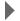Revision history [back]
Hey Evgeny, To actually get the data to disk (for testing) the following python commands are invaluable (I find). This works well for Bruker raw data, if anyone knows the structure of Jeol and Varian files I'm sure I can accommodate. Anyway, after installing numpy for python (numerical-python), code: ### For a 2D [2048, 128] size spectrum >>> import numpy >>> dataSize = 2048 * 128 >>> data = numpy.zeros( dataSize, dtype=numpy.float32 ) ### Set the data to your test values (this sets all to 1.) >>> data = 1. >>> data.tofile( open( 'ser', 'wb'), '', '%f') Writes the data to 'ser'. Note that the data is 32 bit float (can be 32 int) - the numpy default on modern computers is 64 bit (and Bruker data is NOT!). This also produces little endian data, and often older Bruker consoles produce big endian data. You can easily swap the endianess using 'swap' from Wayne Boucher's Azara suite. Do let me know if this was helpful! Thanks, Daniel O'Dononvan2 No.1 Revision posted Aug 05 '10 at 08:55Evgeny Fadeev 577●1

Hey Evgeny,

To actually get the data to disk (for testing) the following python commands are invaluable (I find). This works well for Bruker raw data, if anyone knows the structure of Jeol and Varian files I'm sure I can accommodate. Anyway, after installing numpy for python (numerical-python), code:

``````### For a 2D [2048, 128] size spectrum

spectrum
>>> import numpy
>>> dataSize = 2048 * 128
>>> data = numpy.zeros( dataSize, dtype=numpy.float32 )

)
### Set the data to your test values (this sets all to 1.)

1.)
>>> data = 1.
>>> data.tofile( open( 'ser', 'wb'), '', '%f')

'%f')
``````

Writes the data to 'ser'. Note that the data is 32 bit float (can be 32 int) - the numpy default on modern computers is 64 bit (and Bruker data is NOT!). This also produces little endian data, and often older Bruker consoles produce big endian data. You can easily swap the endianess using 'swap' from Wayne Boucher's Azara suite. Do let me know if this was helpful!

Thanks, Daniel O'Dononvan